# Python tkinter scale

Displaying a scale .
Basics of Python Tkinter

Tkinter Scale to set and get value by moving slider with orient & other options and methods
``````import tkinter as tk
my_w = tk.Tk()
my_w.geometry("200x200")
my_w.title("Scale")
l1=tk.Label(my_w,text="Scale")
l1.grid(row=1,column=1)

my_scale = tk.Scale(my_w, from_=0, to=100, orient='horizontal')
my_scale.grid(row=2,column=1)

my_w.mainloop())``````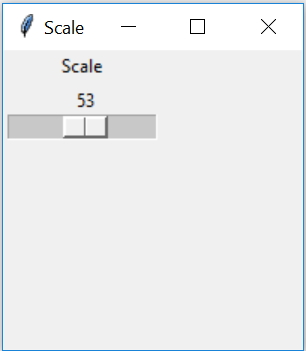Reading value of a scale using get()
``my_scale.get()``
Setting value of a scale using set()
``my_scale.set()``

## Copy value from one scale to other by button click

By using a button click we will assign the value of 2nd scale by reading value of 1st.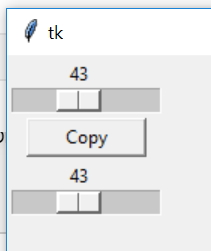``````import tkinter as tk
my_w = tk.Tk()
my_w.geometry("500x500")

def my_upd():
my_scale2.set(my_scale1.get()) # read and set value

my_scale1 = tk.Scale(my_w, from_=0, to=100, orient='horizontal')
my_scale1.grid(row=1,column=1)

my_scale2 = tk.Scale(my_w, from_=0, to=100, orient='horizontal')
my_scale2.grid(row=3,column=1)

b1 = tk.Button(my_w, text='Copy', width=10,command=lambda: my_upd())
b1.grid(row=2,column=1)

my_w.mainloop()``````

## Direct Copy from one Scale to other without any button click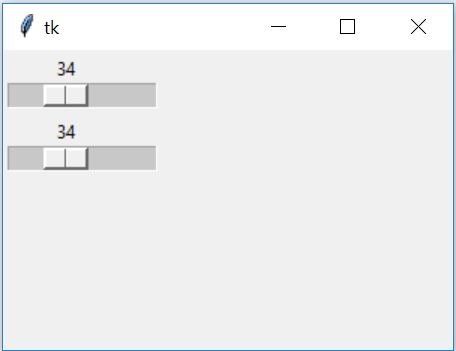``````import tkinter as tk
from tkinter import *
my_w = tk.Tk()
my_w.geometry("300x200")

def my_upd(value):
my_scale2.set(my_scale1.get())

my_scale1 = tk.Scale(my_w, from_=0, to=100, orient='horizontal',command=my_upd)
my_scale1.grid(row=1,column=1)

my_scale2 = tk.Scale(my_w, from_=0, to=100, orient='horizontal')
my_scale2.grid(row=3,column=1)

my_w.mainloop()``````

## Inter connecting Spinbox and Scale widgets

We can add one Spinbox widget and link it to a Scale. Here both are connected so changes in any one will reflect in both.
We used one common StringVar sv here.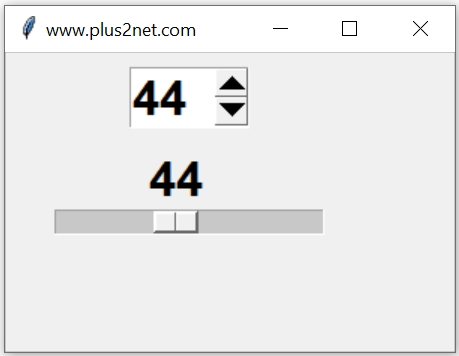``````import tkinter as tk
from tkinter import *
my_w = tk.Tk()
my_w.geometry("300x150")  # Size of the window

sv = StringVar() #string variable
sb = Spinbox(my_w,textvariable=sv, width=15,from_=0,to=100)

sc = Scale(my_w, from_=0, to=100,orient=HORIZONTAL,variable=sv)

my_w.mainloop()  # Keep the window open``````

## Enable disable scale

We can set the option state to active, disabled & normal
``````sv = StringVar() #string variable
sc = Scale(my_w, from_=0, to=100,orient=HORIZONTAL,
state='disabled',variable=sv)
By using config() we can change the state also.
``sc.config(state='normal')``

## Options

We can use these options for configuration of Scale.

## activebackground

Colour of the scale ( handle ) when mouse is over it.

## bg

Background color of the scale area.

## bd

Width of the border

## command

Function we can use when value is changed. ( see the examples above )

## cursor

Shape of the cursor when mouse is over it. For a list of available shapes check the end of Button tutorial.
``font1=('times',18,'bold') # set font style``
``````sc = Scale(my_w, from_=0, to=100,variable=sv,orient='horizontal',
activebackground='red',bg='yellow',bd=5,fg='green',font=font1,
cursor='cross')``````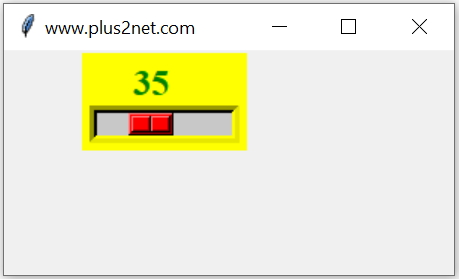## length

Dimension of the slider, it is x value if orientation is horizontal, otherwise y value of vertical orientation.
``````sc = Scale(my_w, from_=1, to=200,variable=sv,
orient='horizontal', length=180)``````

## orient

Orientation of the scale, values can be horizontal or vertical. Default is vertical. See examples above.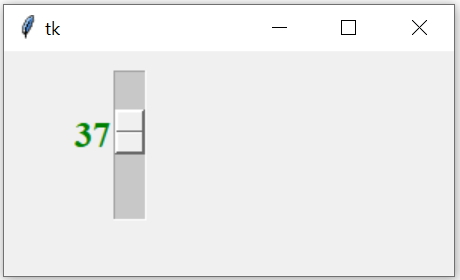## relief

Style of the border. Check the button section for more about relief.
It can take these values raised , sunken ,flat, ridge, solid & groove
``````sc = Scale(my_w, from_=1, to=200,variable=sv,
orient='horizontal',length=180, relief='sunken')``````

## sliderlength

The length of the slider
``````sc = Scale(my_w, from_=0, to=100,variable=sv,
orient='horizontal',sliderlength=10)``````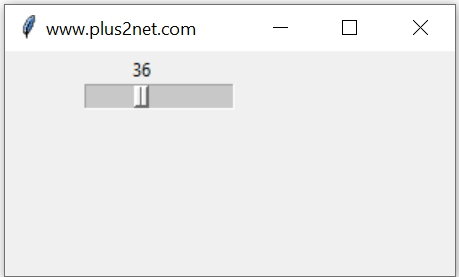## showvalue

Set it to 0 if you don't want the slider value to be displayed.

## state

Can be disabled or enable using this option. See the example above.

## takefocus

Set to to 0 if you don't want it to be on focus through tab cycle along with other widgets.

## width

Default value is 15 pixel, Check the width in different orientation. ( see examples above )

## Exercise

By using a pair of radio buttons, change the state of a scale.

## Subscribe

* indicates required
Subscribe to plus2netplus2net.com